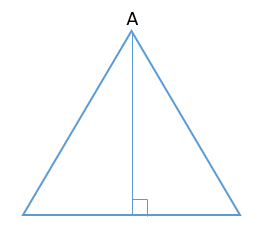# CAT 2020 Question Paper | Quants Slot 2

###### CAT Previous Year Paper | CAT Quants Questions | Question 2

Geometry is the second most important topic of the Quantitative Ability section of the CAT exam. Acing this topic requires you to understand the basic concepts and practice a lot of questions levelling up your preparation game. One of the important things to cover for this are the CAT previous year paper that help you get a hang of the type and pattern of the questions the CAT Question Paper throws at you. Don't wait for you to complete your syllabus before diving into these actual CAT Exam questions. Try this geometry question taken from CAT 2020 Question paper right now without having a look at the solution provided and see where you stand.

Question 2 : From the interior point of an equilateral triangle, perpendiculars are drawn on all three sides. The sum of the lengths of the perpendiculars is 's'. Then the area of the triangle is

1. $$frac{s^{2}}{2√3}$ 2. $\frac{2s^{2}}{√3}$ 3. $\frac{s^{2}}{√3}$ 4. $\frac{√3s^{2}}{2}$ ## 🎉Get Upto ₹10,000 off on our CAT 23/24 courses.🎉Get flat ₹6,000 off on IPM courses.Valid till 30th September 2023! #### ★Register Now! ### Video Explanation ## Best CAT Coaching in Chennai #### CAT Coaching in Chennai - CAT 2022Limited Seats Available - Register Now! ### Explanatory AnswerFrom any point on a equilateral triangle, if we draw three perpendiculars on other sides Those three perpendicular will add up to = Altitude of an equilateral triangle We take the point to be A, and try to draw 3 perpendiculars$Can draw only one)
And is same as altitude of the equilateral triangle
So, s = $$frac{√3}{2}$ a --> a = $\frac{2s}{√3}$ By substituting the value in Area of an equilateral triangle $\frac{√3}{4}$a2 $\frac{√3}{4}$ ×$$$frac{2s}{√3}$)2= $\frac{s^{2}}{√3}$ The question is "From the interior point of an equilateral triangle, perpendiculars are drawn on all three sides. The sum of the lengths of the perpendiculars is 's'. Then the area of the triangle is" ##### Hence, the answer is, "$\frac{s^{2}}{√3}$" ###### 🎉Get Upto ₹10,000 off on our CAT 23/24 courses.🎉Flat ₹6000 off on IPM courses. ###### Prepare for CAT 2023 with 2IIM's Daily Preparation Schedule ###### Know all about CAT Exam Syllabus and what to expect in CAT ###### Already have an Account? ###### CAT Coaching in ChennaiCAT 2023 Classroom Batches Starting Now! @Gopalapuram ###### Best CAT Coaching in Chennai Introductory offer of 5000/- Attend a Demo Class ###### Best Indore IPM & Rohtak IPM CoachingSignup and sample 9 full classes for free. Register now! ##### Where is 2IIM located? 2IIM Online CAT Coaching A Fermat Education Initiative, 58/16, Indira Gandhi Street, Kaveri Rangan Nagar, Saligramam, Chennai 600 093 ##### How to reach 2IIM? Mobile:$91) 99626 48484 / 94459 38484
WhatsApp: WhatsApp Now
Email: info@2iim.com